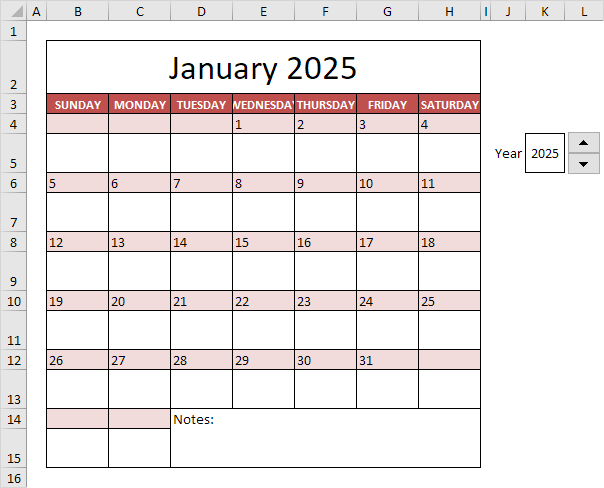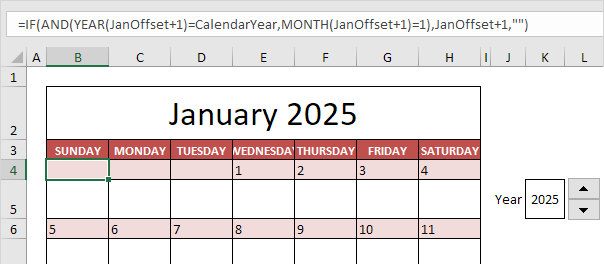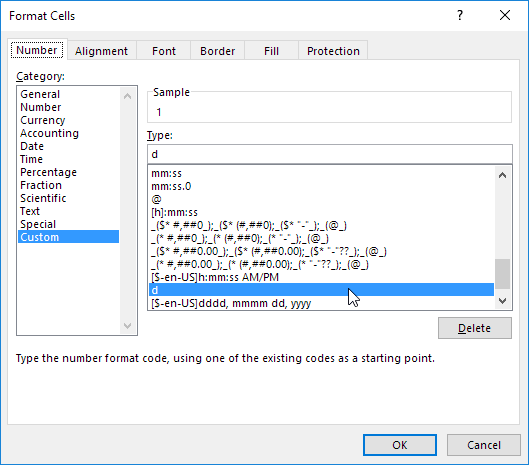# Calendar

This example describes how to create a calendar in Excel (2023 calendar, 2024 calendar, etc). If you are in a hurry, simply download the Excel file.

Note: the instructions below do not teach you how to format the worksheet. We assume that you know how to change font sizes, font styles, insert rows and columns, add borders, change background colors, etc.

This is what January 2025 looks like.The big question is: how do we tell Excel that January 2025 starts on a Wednesday?

1. First, to make the formulas easier to understand, we created the following named ranges.

Name Cells
CalendarYear K5
JanOffset =DATE(CalendarYear,1,1)-WEEKDAY(DATE(CalendarYear,1,1))

Explanation: dates are stored as numbers in Excel and count the number of days since January 0, 1900. The DATE function returns the number that represents the date. The DATE function accepts three arguments: year, month and day. The WEEKDAY function returns a number from 1 (Sunday) to 7 (Saturday) representing the day of the week of a date. For example, January 1st, 2025 is the same as 45658. This date falls on a Wednesday. In other words, =DATE(CalendarYear,1,1) equals 45658 and WEEKDAY(DATE(CalendarYear,1,1)) equals 4. As a result, JanOffset equals 45658 - 4 = 45654.

2. Let's take a look at the formula in cell B4.Explanation: JanOffset+1 is nothing more than a date. In fact, it is (4-1) days earlier than January 1st, 2025. This formula checks if the year of this date equals CalendarYear and the month equals January (1). If true, it returns the date. If not, it returns an empty string ("").

3. Let's take a look at the formula in cell E4.Explanation: JanOffset+4 is nothing more than a date. In fact, it is (4-4) days earlier than January 1st, 2025. This is the first day of the year! This formula checks if the year of this date equals CalendarYear and the month equals January (1). This is true! Excel returns the date.

4. We changed the format to "day format" to only display the day of the month.Note: in a similar way, you can create the formulas for the other days in January and the other months. This calendar tool lets you create a calendar for every year you want! Just use the Spin Button to increase the calendar year.

Go to Next Chapter: Data Validation